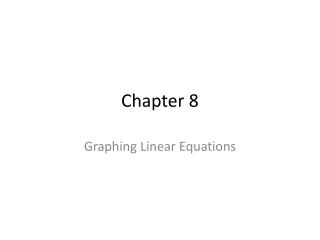DownloadDownload PresentationChapter 8

# Chapter 8

Download Presentation## Chapter 8

- - - - - - - - - - - - - - - - - - - - - - - - - - - E N D - - - - - - - - - - - - - - - - - - - - - - - - - - -
##### Presentation Transcript

1. Chapter 8 Graphing Linear Equations

2. §8.1 – Linear Equations in 2 Variables • What is an linear equation? What is a solution? 3x = 9 • What is an linear equation in 2 variables? What is a solution? 2x + y = 10

3. §8.1 – Linear Equations in 2 Variables • Linear equations in 2 variables • Ordered pairs • Complete ordered pair given one of the values • Rectangular coordinate system structure • Axes and quadrants • Plotting points • Determining coordinates of plotted points

4. §8.2 – Graphing Linear Equations • Algorithm on p. 285 • Find 3 solutions • Plot corresponding points • Draw line thru points • If not straight – find your error • Ex: Graph 3x + 2y = 12 • x and y intercepts

5. §8.2 – Graphing Linear Equations • Horizontal lines y = a • Vertical lines x = b • Problem #42 p. 290 • Why only positive values?

6. §8.3 – The Slope of a Line • What is slope? • Slope formula: • General concepts • Positive slope • Negative slope • 0 slope • Undefined slope

7. §8.3 – The Slope of a Line • Slope – intercept form: • General concepts • y isolated • m is the slope • b is the y–intercept

8. §8.3 – The Slope of a Line • For each of the following lines, find the slope, the y–intercept, and graph the line

9. §8.3 – The Slope of a Line • Parallel lines • What are they? • Same slope • Problems 8.3 #31, 32 (p. 297) – just parallel • Perpendicular lines • What are they? • Slopes are negative reciprocals of each other • Problems 8.3 #31, 32 (p. 297) – just perpendicular

10. §8.4 – The Equation of a Line • Slope – intercept form: • Find equation given m and b • Problem 8.4 #22 (p. 301) • Point – Slope form: • Find equation given point and slope • Problem 8.4 #32 (p. 301) • Find equation given 2 points • Problem 8.4 #52 (p. 301)